# PSAT Math : How to find the equation of a parallel line

## Example Questions

### Example Question #1 : Coordinate Geometry

Which of the following is the equation of a line that is parallel to the line 4x – y = 22 and passes through the origin?

y – 4x = 22

4x = 8y

(1/4)x + y = 0

4x + 8y = 0

4x – y = 0

4x – y = 0

Explanation:

We start by rearranging the equation into the form y = mx + b (where m is the slope and b is the y intercept); y = 4x – 22
Now we know the slope is 4 and so the equation we are looking for must have the m = 4 because the lines are parallel. We are also told that the equation must pass through the origin; this means that b = 0.

In 4x – y = 0 we can rearrange to get y = 4x. This fulfills both requirements.

### Example Question #2 : Coordinate Geometry

What line is parallel to 2x + 5y = 6 through (5, 3)?

y = –2/5x + 5

y = 3/5x – 2

y = 5/3x – 5

y = 5/2x + 3

y = –2/3x + 3

y = –2/5x + 5

Explanation:

The given equation is in standard form and needs to be converted to slope-intercept form which gives y = –2/5x + 6/5. The parallel line will have a slope of –2/5 (the same slope as the old line). The slope and the given point are substituted back into the slope-intercept form to yield y = –2/5x +5.

### Example Question #3 : Coordinate Geometry

What line is parallel to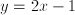through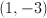?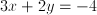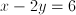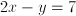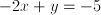Explanation:

The slope of the given line is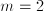and a parallel line would have the same slope, so we need to find a line throughwith a slope of 2 by using the slope-intercept form of the equation for a line.  The resulting line is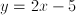which needs to be converted to the standard form to get.

### Example Question #1 : How To Find The Equation Of A Parallel Line

Find the equation of a line parallel to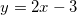that also passes through the point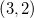.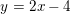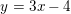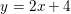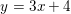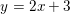Explanation:

Since they are parallel, the line will have the same slope as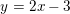.

Thus, it will take the form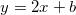.

We then use the point (3,2) to solve for: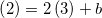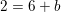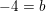so the equation of the line is### Example Question #2 : How To Find The Equation Of A Parallel Line

In the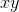-plane, the line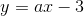is parallel to the line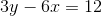. What is the value of?Explanation:

In this equation,is equal to the slope of the line.  You have been given a line parallel to the line containing variable, and since parallel lines have the same slope, all you need to do is figure out the slope ofin order to figure out whatis.  To figure out the slope of, you need to convert the equation intoform, which means isolatingon one side of the equation.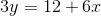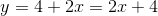Given this solution, the slope of the parallel lines, and thus, is equal to 2.

### Example Question #3 : How To Find The Equation Of A Parallel Line

Which of the following lines has zero points of intersection with the line,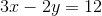?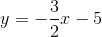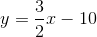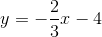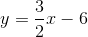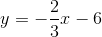Explanation:

This prompt asks you to find a line that is parallel to the one given. Parallel lines have no points of intersection (as opposed to intersecting lines which have 1 point of intersection). Parallel lines have the same slope, but different y-intercepts. If they had the same y-intercept, then they would actually be the same line.

We can find the slope of the first line by converting it to slope-intercept form.First, subtract 3x from both sides.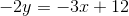Then, divide both sides by -2.The slope of the line is 3/2. The only answer that has that same slope, but a different y-intercept is.